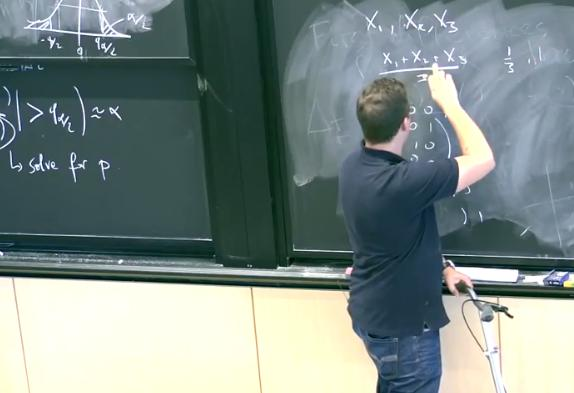# InfoCoBuild

## 18.650 Statistics for Applications

18.650 Statistics for Applications (Fall 2016, MIT OCW). Instructor: Professor Philippe Rigollet. This course offers an in-depth the theoretical foundations for statistical methods that are useful in many applications. The goal is to understand the role of mathematics in the research and development of efficient statistical methods. (from ocw.mit.edu)Lecture 01 - Introduction to Statistics Lecture 02 - Introduction to Statistics (cont.) Lecture 03 - Parametric Inference Lecture 04 - Parametric Inference (cont.) and Maximum Likelihood Estimation Lecture 05 - Maximum Likelihood Estimation (cont.) Lecture 06 - Maximum Likelihood Estimation (cont.) and the Method of Moments Lecture 07 - Parametric Hypothesis Testing Lecture 08 - Parametric Hypothesis Testing (cont.) Lecture 09 - Parametric Hypothesis Testing (cont.) Lecture 10 Lecture 11 - Parametric Hypothesis Testing (cont.) and Testing Goodness of Fit Lecture 12 - Testing Goodness of Fit (cont.) Lecture 13 - Regression Lecture 14 - Regression (cont.) Lecture 15 - Regression (cont.) Lecture 16 Lecture 17 - Bayesian Statistics Lecture 18 - Bayesian Statistics (cont.) Lecture 19 - Principal Component Analysis Lecture 20 - Principal Component Analysis (cont.) Lecture 21 - Generalized Linear Models Lecture 22 - Generalized Linear Models (cont.) Lecture 23 - Generalized Linear Models (cont.) Lecture 24 - Generalized Linear Models (cont.)

 References 18.650 Statistics for Applications, Fall 2016 Instructor: Professor Philippe Rigollet. Lecture Notes. Assignments: Problem Sets (no solution). This course offers an in-depth the theoretical foundations for statistical methods that are useful in many applications.